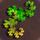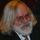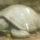## General Question# If you know the values of a function at the three points of a triangle, how can I determine the values at points in the interior? {details}?

Asked by PhiNotPi (12681) July 28th, 2012

There is a triangle ABC. The coordinates of each of the corners are known. In addition, each corner is given an extra value, which is known. Given any two points, the midpoint between them is given the value that is the average of those two points. My goal is to find a way to calculate the values of points directly, given the coordinate of the point.

Here is an illustration I made to show you what I am talking about. The images to the left show that all of the points on the blue lines should all have the same values. On the right, you can see one method of solving a triangle when the three corners are not the same. However, you can see that this process may take an unlimited number of iterations to solve for any particular point.

How do I find the values of any particular point directly, taking the same amount of time for each point?

Observing members: 0Composing members: 0Noticing the fact that on the first two triangles in the image that points of equal values lay on parallel lines, I wonder if this is the case for the larger triangle. If it is, then the parallel lines are not parallel to the sides of the triangle, and I wonder how to find the equations for the lines.

PhiNotPi (12681)“Great Answer” (0) Flag as…It seems to me that you are working with barycentric coordinates. Given 3 points A, B and C with corresponding values of v1, v2 and v3, any point in the triangle can be expressed as pA + qB + rC, where p+q+r =1 and p>0, q>0 and r >0. The associated value at the point is pv1 + qv2 +rv3. I don’t know of any way of converting from Cartesian to barycentric coordinates other than setting up the obvious 3 linear equations and solving for p, q and r. Check here I just looked at it briefly. It seems that the problem can be reduced to solving two equations.

LostInParadise (30456)“Great Answer” (3) Flag as…The equation is a simple linear equation in two variable. F(x,y) = ax +by +c
You have three boundary values (the three points on the triangle.)
Substitute the values at the ponts:
F(P1)=a(P1.X)+b(P1.Y)+c
F(P2)=a(P2.X)+b(P2.Y)+c
F(P3)=a(P3.X)+b(P3.Y)+c
Solve for a,b, and c.
Now you have an equation that will take any x and y and give you F(x,y)
F(x,y) = ax +by +c

Grisson (4634)“Great Answer” (1) Flag as…I think it has to be a plane. 3 non-co-linear points define a plane so solve for the plane and you got it.

roundsquare (5522)“Great Answer” (0) Flag as…Triangle impies plane.

Grisson (4634)“Great Answer” (0) Flag as…or